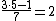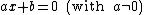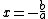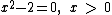symbolic computing

symbolic computing

[sim¦bäl·ik kəm′pyüd·iŋ]
(computer science)
The development and use of symbolic systems.
McGraw-Hill Dictionary of Scientific & Technical Terms, 6E, Copyright © 2003 by The McGraw-Hill Companies, Inc.

Symbolic computing

The process of manipulating numbers and variables according to the rules of mathematical logic. Variables are used to represent a real number or a set of real numbers to exact precision. Numbers can be added, subtracted, multiplied, and divided. Numerical calculations or numerical computations involve performing these operations. For example, if the symbol * is used to represent multiplication and / is used to represent division, then the equationrepresents a numerical computation whose result is 2. In mathematics, it is also possible to use symbols to represent numbers and to do computations with the symbols. For example,can also be expressed asIn this calculation or computation, the mathematical rules for subtraction and division are obeyed. The symbol x is treated as an unknown or variable that is to be solved for, and a and b as parameters representing unknown but fixed numbers.

In the 1990s, mathematical software packages became available that could manipulate symbols according to the rules of mathematics. They are also called symbolic computational packages and are programming environments, since any real number has a decimal expansion. These packages have the ability to obtain exact answers as symbols. For example, ifwere entered into a symbolic computation package, the software package would return the answerThe software package also allows the user to then ask for an approximation to 2 to any number of digits. The decimal units for 2 are determined using an algorithm that performs numerical calculation (called a numerical algorithm). Symbolic computation packages allow both symbolic and numerical computation. See Algorithm, Computer programming, Mathematical software

The capability of small computers and hand-held calculators to do extremely complicated calculations in a small amount of time led to the development of mathematical software packages that could do symbolic manipulation. These symbolic manipulation packages (also called computer algebra systems or symbolic computation systems) can do algebra, trigonometry, number theory, calculus, ordinary and partial differential equations, matrix algebra, and other areas of mathematics by manipulating the symbols according to the rules of mathematics much faster than armies of mathematicians. The answers are given exactly in symbolic form and can then be approximated numerically using routines in the package.

The advent of visualization on the computer has made it possible for these packages to plot data or functions in many forms. The functions are entered symbolically, and the software evaluates them numerically so that numbers can be plotted. The graphs generated by the plot routines can be labeled, rotated, or animated, among many other possibilities.

Hand-held calculators can perform symbolic manipulation, graph data or functions, and be programmed to carry out a sequence of operations. Mathematical software packages have been developed that can perform symbolic manipulation, graph data or functions, and be programmed using routines in the package. These packages run on most commonly used operating systems. They can also be called by computer programs to perform manipulations in the program. There are packages that do statistics, computational finance, computational physics, and computational chemistry. See Operating system, Programming languages

McGraw-Hill Concise Encyclopedia of Engineering. © 2002 by The McGraw-Hill Companies, Inc.
Mentioned in ?
References in periodicals archive ?
For upper level undergraduates in physics and engineering, and for graduate students and professionals seeking reinforced knowledge of symbolic computing.,Differing from other texts, this book ties the mathematical topics under consideration to symbolic computation, which, the author asserts, can enhance understanding, help with visualization of results, and out-do purely numerical approaches when applied to real-world problems.
Systems that address these two canonic problems or any related ones cover the whole spectrum between: (1) symbolic computing vs.
The application combines a symbolic computing engine and traditional numeric solvers with the simulation and modeling process.
Maplesoft (Ontario, Canada) introduces MapleSim[TM], a multi-domain modeling and simulation tool in which the world's most advanced symbolic computing engine comes together with traditional numeric solvers to supercharge the simulation and modeling process.
This complement to the proceedings for the 2006 International Conference on Principles and Practice of Constraint Programming includes selected papers from a range of lectures and workshops about gathering, exploiting and unifying ideas shared by operations research, numerical analysis, symbolic computing, scientific computing, artificial intelligence and programming languages.
* Importing of formulas directly into symbolic computing systems.
Barry Adams, a researcher at Laurentian Univ., Sudbury, Ontario, uses the Maple symbolic computing algebra system to calculate complex energy corrections for hydrogen-based systems in external field potentials.
ICOT is one of only a few sites in the world that is studying massively parallel symbolic computing.